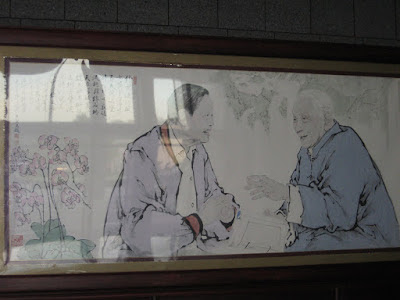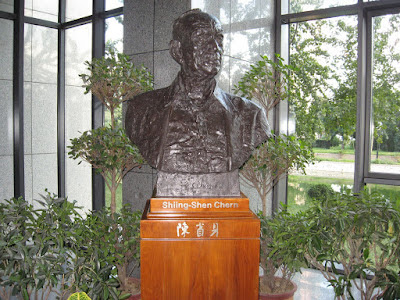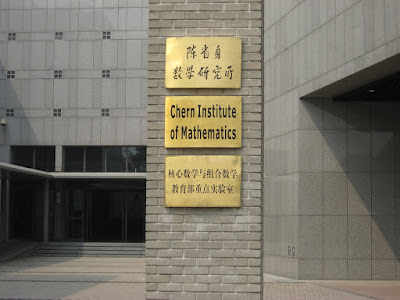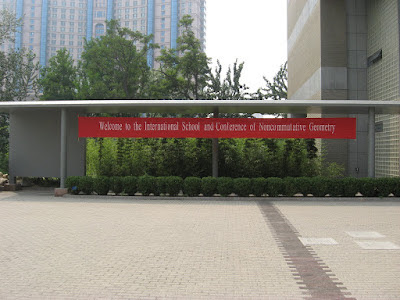## Thursday, December 6, 2007

### Noncommutative Geometry in China

During August 15-30, 2007, a school and workshop on noncommutative geometry took place at the Chern Institute in Nankai University in Tianjin, China. The first two weeks and part of the third week was devoted to a series of mini-courses (5 lectures each) on various aspects of noncommutative geometry. This conference and its success owes a lot to the Chern Institute and to our Chinese friends on the organizing committee. We really had a very good time in Tianjin. A big thank you to all for their hospitality and efforts! Here is a list of mini-courses and speakers:

G. Yu: Cyclic cohomology and Connes-Chern character;
G. Gong: Operator algebras and K-theory;
L. Guo: Rota-Baxter algebra with applications to renormalizations;
A. Thom: L^2-Betti numbers for von Neumann algebras;
T. Natsume: Index theory and noncommutative geometry;
V. Mathai: Geometry of the determinant line bundle in noncommutative geometry;
W. van Suijlekom: Noncommutative geometry and physics;
M. Khalkhali: Noncommutative geometry and the local index formula

The first two mini-courses were meant to warm up the audience of mostly grad students and postdocs for lectures in the next weeks. I asked the mini-course speakers to write a blurb on what they talked and most of them budged. So here are their reports and my apologies for delaying this so long!

Andreas Thom; L^2-invariants and von Neumann algebras:
This mini-course consisted of five lectures around the algebraic theory of l^2-invariants for groups and tracial algebras. After a review of the basic tools of homological algebra and an introduction to Lueck's dimension theory for modules over finite von Neumann algebras, a new and conceptual proof of Gaboriau's theorem about proportionality of l^2-Betti numbers for measure equivalent groups was presented, see (L^2-invariants and rank metric, arXiv:math/OA.0607263). In the third and fourth lecture recent work of Peterson-Thom (Group cocycles and the ring of affiliated operators, arXiv:0708.4327) was presented. Here, the focus was on applications to geometric and combinatorial group theory. Indeed, as was shown in the course, non-vanishing of the first l^2-Betti number of a group implies strong restrictions on the subgroup structure. Several conjectures related to Atiyah's conjecture were discussed in detail. In the fifth lecture, a quick review of the work of Connes-Shlyakhtenko (math.OA/0309343) on l^2-invariants for tracial algebras was given.

Walter van Suijlekom; Noncommutative geometry and physics:
The subject of my lectures in Tianjin was the noncommutative geometry of the Standard Model of high-energy physics. We started in the first two lectures with an overview of the essential parts of the Standard Model in order to recognize it later on when derived in the setting of noncommutative geometry.In the remaining three lectures, we looked at how the so-called Spectral Action Principle allows us to obtain the full (Lagrangian of the) Standard Model from a natural noncommutative geometry given as a real spectral triple. We based our lectures on the book ``Noncommutative Geometry, Quantum Fields. and Motives'' by Connes and Marcolli, appearing at the beginning of 2008.

Toshi Natsume; Index theory and noncommutative geometry:
in this short course historical background was given, and the meaning and basic properties of the index were explained. In fact the Atiyah-Singer Index Theorem holds on all sorts of manifolds, but for this course we focused on elliptic operators on Euclidean space, and proved the index theorem here (still a highly nontrivial result). The course required background in multivariable calculus and linear algebra. An introductory survey of the additional tools (from functional analysis and topology) needed to prove the theorem were given. The Atiyah-Singer Index Theorem opened the door to a new world of interaction between different areas of mathematics, where analytic machinery such as operator algebras can play a significant role in topology and geometry. A crystallization of this idea is noncommutative geometry, currently important particularly in today’s mathematical physics. The course was concluded by explaining some fundamental ideas and remarkable results in noncommutative geometry

Li Guo; Connes-Kreimer algebraic Birkhoff decomposition and applications:
The algebraic Birkhoff decomposition of Connes
and Kreimer is a fundamental result in their Hopf algebra
approach to renormalization of perturbative quantum field
theory. We provide enough background to present this result
and prove it in the context of Rota-Baxter algebras through
Spitzer's identity and Atkinson factorization. We then illustrate
its applications to renormalization in quantum field theory
and in multiple zeta values.

Masoud Khalkhali; Noncommutative geometry and the local index formula:
This was an introduction to the local index formula of Connes and Moscovici (GAFA 95), currently the most general and most elaborate form of an index theorem available in NCG. To understand the theorem, almost all of the key ideas of NCG must be introduced: K-theory and K-homology, cyclic cohomology, index pairing, Connes-Chern character maps, Dixmier trace and spectral zeta functions, and noncommutative residues. As such I found the topic an ideal way of introducing these concepts and tools all with the goal of reaching to one of the summits of NCG.

Here are a few pictures I took in TianjinChairman Mao still tries to lead! (why not?)A nice painting depicting old friends S. S. Chern and C. N. Yang in the lobby of the Chern Institute. ( I can only imagine what they are talking about......but your `gauge fields' are much the same as my `connections' and your `field strengths' are like my `curvatures'........)Long Live Noncommutative Geometry!